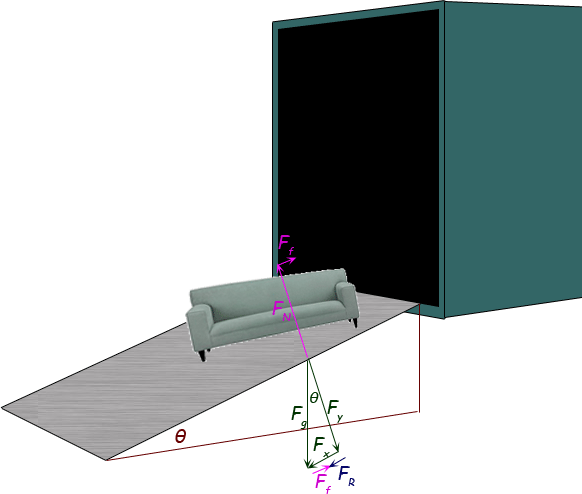SEARCH HOMEMath Central Quandaries & QueriesQuestion from William, a student: A couch with a mass of 1 X 10^2kg is placed on an adjustable ramp connected to a truck.  As one end of the ramp is raised, the couch begins to move downward.  If the couch slides down the ramp with an acceleration of .70 meters per second when the ramp angle is 25 degrees, what is the coefficient of kinetic friction between the ramp and couch? I drew a force diagram and if I did it correctly I identified the forces involved as "mg" (mass x gravity), "Fn" (normal force) and the "Ff" (frictional force).  I know that we have the couch sliding down the ramp a .70 m/s but I don't think this a force and I'm not sure how this info fits into the problem.  I know that the formula for calculating the coefficient of friction is Ff/Fn.  Based upon the force diagram I drew and calculated Fn to be 1082N.  I can't seem to get past this point.  How do I determine what the frictional force is?Hi William.

I've drawn the scene, forces and force components below.First, I hope you can recognize that the angle θ for the ramp is the same as the angle θ between the force due to gravity (Fg ) and its component perpendicular to the ramp (Fy ). This is due to similar triangles.

Now, you want the co-efficient of kinetic friction and that is μ in the equation Ff = μ FN, where Ff is the force of friction and FN is the normal force. Thus we have:

μ = Ff / FN

You know that the magnitude of the normal force FN is the same as the magnitude of the force Fy (otherwise the couch would be sinking into the ramp or levitating!) But right triangle trigonometry tells us that Fy = Fg cos θ. So:

μ = Ff / ( Fg cos θ)

I've transcribed the frictional force from the top of the diagram to the bottom so you can see how it has to add up. The resultant force (giving rise to the acceleration of the couch) is FR. This is a result of the component of the gravitational force that is parallel to the ramp (Fx ) and the frictional force that opposes it (Ff ). So (using magnitudes only), Ff = Fx - FR . This means:

μ = (Fx - FR ) / ( Fg cos θ)

Again using standard right triangle geometry, we know that Fx = Fg sin θ, so:

μ = ( Fg sin θ - FR ) / ( Fg cos θ)

By Newton's Law, the resultant force FR = maR , where aR is the resultant acceleration. Thus,

μ = ( Fg sin θ - maR ) / ( Fg cos θ)

And lastly, Fg is simply mg, so the mass cancels out and our final equation is:

μ = ( g sin θ - aR ) / ( g cos θ)

So from here, you have all the values you need. Note that the question says the resultant acceleration is 0.70 meters per second [sic]. Meters per second isn't an acceleration, so something is wrong with the question. My guess is this is a typo and it should say 0.70 meters per second2 .

Hope this helps,
Stephen La Rocque.Math Central is supported by the University of Regina and The Pacific Institute for the Mathematical Sciences.